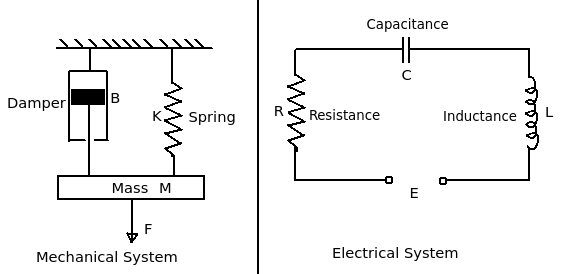# Corresponding factor for displacement of mass attached to spring

Q.  The displacement of a mass attached to a spring in mechanical system is corresponding to _______ in the electrical system
- Published on 15 Sep 15

a. inductance
b. resistance
c. capacitance
d. current

#### Discussion

• Pankaj Chaugule   -Posted on 24 Nov 15- Considering above diagrams of a mechanical system and electrical system, characteristic equation for each system can be derived.

- The characteristic equation for mechanical system is given by,

M(d2x/dt2) + B(dx/dt) + Kx = F …..........................(1)

Where,

M(d2x/dt2) = Inertia force

B(dx/dt) = Damping force

Kx = Spring force

- Whereas, the characteristic equation for electrical system is given by,

L(d2i/dt2) + R(di/dt) + (1/C)i = Eo cos ωt ….........................(2)

Where,

L(d2i/dt2) = Voltage across inductance

R(di/dt) = Voltage across resistance

(1/C)i = Voltage across capacitance

From above equations (1) and (2), we can say that the displacement (x) of a mass attached to a spring in mechanical system is corresponding to current (i) in the electrical system.

## ➨ Post your comment / Share knowledgeEnter the code shown above:

(Note: If you cannot read the numbers in the above image, reload the page to generate a new one.)